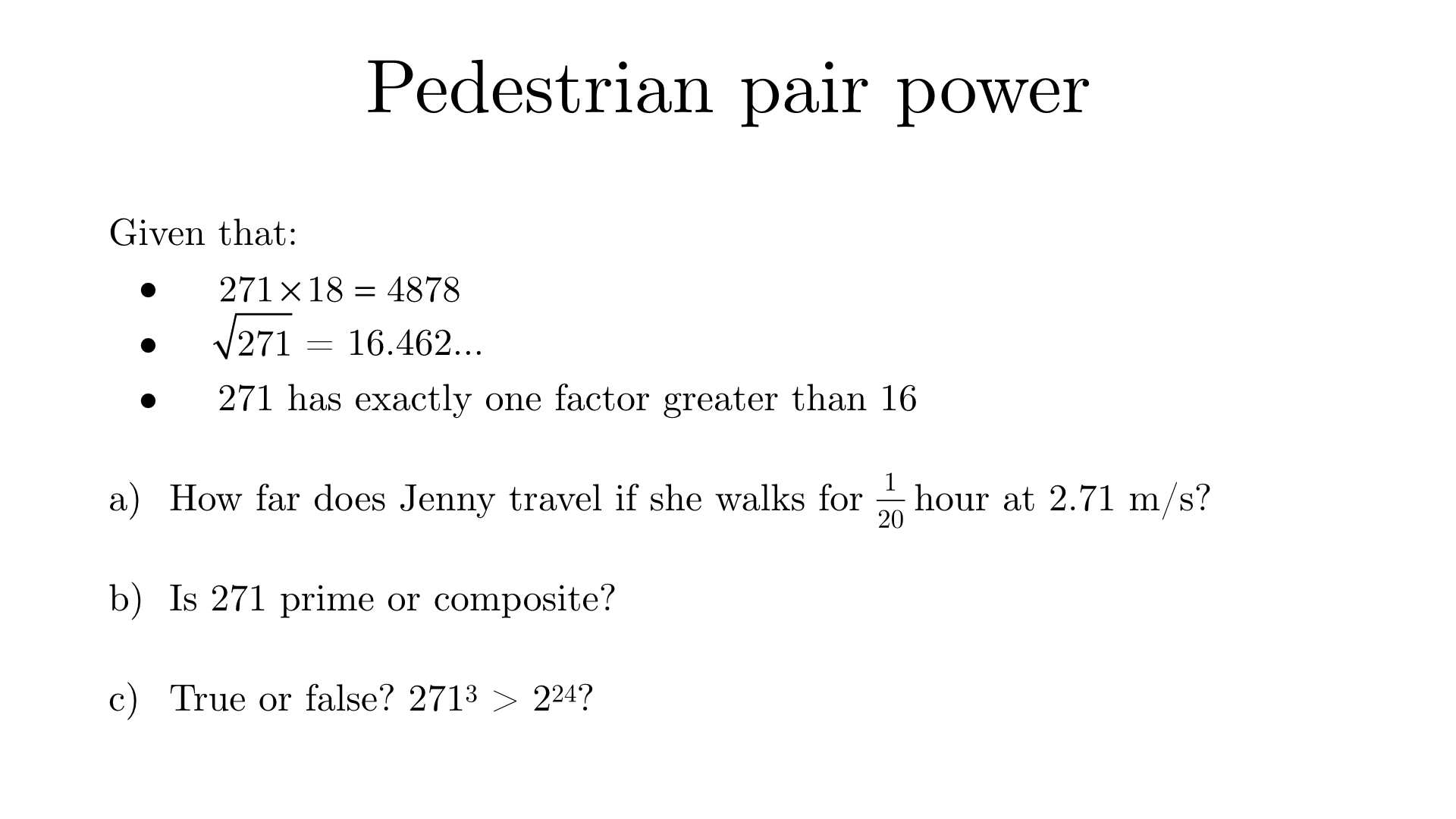Solution to (a)
$$\frac{1}{20}$$ of an hour is $$3$$ minutes, which is $$180$$ seconds. $$2.71 \times 180 = 487.8$$ metres.
Solution to (b)
271 is not a square number, and therefore has an even number of factors. Each factor pair must consist of one factor less than the square root of 271 and one greater. (If both were less, the product would be less than 271; if both were greater, then the product would be greater.) Since 271 has only one factor greater than 16, and this factor is 271 itself, it is prime.
Solution to (c)
We are given that $$\sqrt{271}$$ > $$16$$, so $$271^{\frac{1}{2}}$$ > $$16$$. Raising both sides to the power of 6, we get $$271^{3}$$ > $$16^{6}$$. Since $$16 = 2^{4}$$, we can see that $$271^{3}$$ > $$2^{24}$$, so the inequality given is true.